Kirchhoff formula

Kirchhoff integral

The formula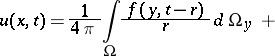(1)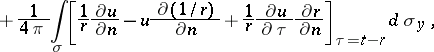expressing the value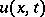of the solution of the inhomogeneous wave equation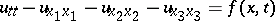(2)

at the pointat the instant of timein terms of the retarded volume potential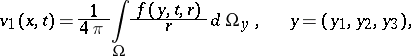with density, and in terms of the values of the function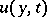and its first-order derivatives on the boundaryof the domainat the instant of time. Hereis a bounded domain in the three-dimensional Euclidean space with a piecewise-smooth boundary,is the outward normal toand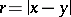is the distance betweenand.

Letwhere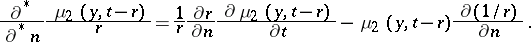The integralsandare called the retarded potentials of the single and the double layer.

The Kirchhoff formula (1) means that any twice continuously-differentiable solutionof equation (2) can be expressed as the sum of the retarded potentials of a single layer, a double layer and a volume potential:In the case when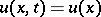and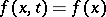do not depend on, the Kirchhoff formula takes the formand gives a solution of the Poisson equation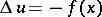.

The Kirchhoff formula is widely applied in the solution of a whole series of problems. For example, ifis the ball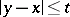of radiusand centre, then formula (1) is transformed into the relation(3)

where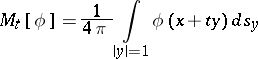is the average value ofover the surface of the sphere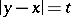,(4)

If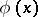andare given functions in the ball, with continuous partial derivatives of orders three and two, respectively, andis a twice continuously-differentiable function for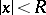,, then the functiondefined by (3) is a regular solution of the Cauchy problem (4) for equation (2) whenand.

Formula (3) is also called Kirchhoff's formula.

The Kirchhoff formula in the formfor the wave equation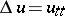(5)

is remarkable in that the Huygens principle does follow from it: The solution (wave)of (5) at the pointof the space of independent variables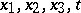is completely determined by the values of,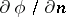andon the spherewith centre atand radius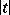.

Consider the following equation of normal hyperbolic type: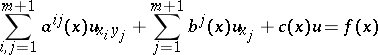(6)

with sufficiently-smooth coefficients,,, and right-hand sidein some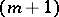-dimensional domain, that is, a form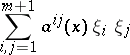that at any point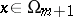can be reduced by means of a non-singular linear transformation to the form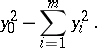The Kirchhoff formula generalizes to equation (6) in the case when the number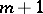of independent variablesis even . Here the essential point is the construction of the function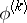that generalizes the Newton potentialto the case of equation (6). For the special case of equation (6),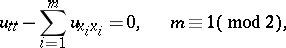(7)

the generalized Kirchhoff formula is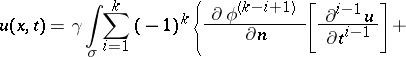(8)whereis a positive number,is the piecewise-smooth boundary of an-dimensional bounded domaincontaining the pointin its interior, andis the outward normal to. Further,and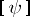denotes the retarded value of:Formula (8) for equation (6) is sometimes called the Kirchhoff–Sobolev formula.